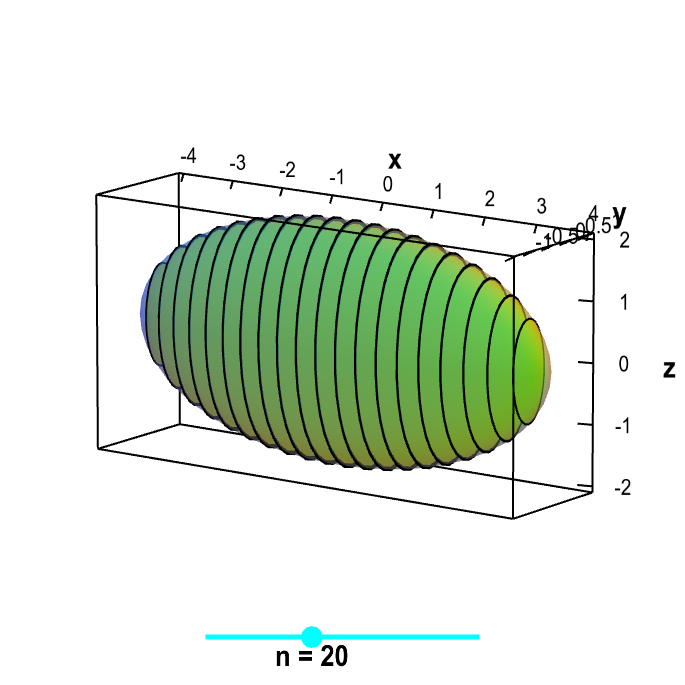# Math Insight

### Applet: Stack of cross sectionsInside the transparent ellipsoidal region $x^2/16+y^2+z^2/4 \le 1$ are a stack of cross sections that are cut perpendicular to the $x$-axis. If you view the $x$-axis as being vertical, then the cross sections are stacked on top of each other. You can drag the cyan point on the slider to change the number of cross sections.